# Never trust a THD+N measurement: v1

Caveat: This is basically a geek version of a cover tune. The point that I make here was one that I originally heard someone else present at an AES convention years ago. However, since I haven’t heard anyone tell this story since, I’ve written it here.

Let’s build two black boxes, each of which creates a measurable distortion. We’ll call them Box “A” and Box “B”.

Box “A” has a measured THD+N of 20%. Box “B” has a measured THD+N of 2%. We’ll be using the old-fashioned way of measuring THD+N where we put a sine wave into the device, and apply a notch filter to the output at the same frequency of the sine wave and find the ratio of the level of the sine wave to the output of the notch filter.

Let’s put a 500 Hz sine wave into the boxes and listen to the output. The original sine wave sounds like the following:

Update Required

The sine wave at the output of Box “A” (with a THD+N of 20%) sounds like the following:

Update Required

The sine wave at the output of Box “B” (with a THD+N of 2%) sounds like the following:

Update Required

So far so good. There should be no surprises yet.

Now let’s put a recording of something that I listen to all the time (my own voice) into the same black boxes to see what happens.

We’ll start with the original recording (this is just a file that I happened to have on my hard drive for testing imaging – ignore the fact that it talks about coming from the left channel only – your computer will probably play it as a mono file out both channels – this is irrelevant to the discussion):

Update Required

Now let’s listen to how that recording sounds at the output of Box “A” (with a measured THD+N of 20%)

Update Required

As you’ll hear, there is no audible distortion on the sound file, despite the fact that it has gone through a box that generates a distortion that we measured to be 20%.

Now let’s listen to how the original recording sounds at the output of Box “B” (with a measured THD+N of 2%)

Update Required

As you will probably hear in that last sound file, the Box “B” – the one with “only” 2% distortion sounds MUCH worse than either the original sound file or the output of Box “A” which should have much more audible distortion.

So, the question is “why?”

Let’s look at the waveforms to see what’s going on here.

The original sine wave looks like the following:

After that sine wave has gone through Box “A”, the output looks like the following:

As you can see, I’ve created Box “A” to generate its distortion by clipping the signal at a limits of -0.5 and 0.5.

The output of Box “B” when fed with the same sine wave looks like the following:

If we zoom in on that plot, it looks like the following:

So, as you can see, I’ve made Box “B” to generate a zero-crossing distortion – but a pretty small one.

The reason the THD+N of Box “A” is 20% and that of Box “B” is only 2% is not just because the “damage” done to the signal is bigger with Box “A”. It’s also caused by where the damage is done. This might not make sense, so let’s look at the signals  a little differently.

Let’s do a histogram of the original sine wave. This tells us how often the sample values are a given value. This is shown below in the following plot.

This histogram shows that the sample values in the original sine wave are usually near -1 and +1, and rarely around 0.

Now let’s look at a histogram of the output of Box “A” – the distorted sine wave with 20% THD+N. It looks like the following:

As can be seen in the plot above, the sample values from the original sine wave that were below -0.5 are now all congregated at -0.5, and the values that were above 0.5 are now congregated at 0.5. This is the result of the clipping applied to the signal.

By comparison, the histogram of the output of Box “B” is shown below:

As you can see by comparing these last two plots, the zero crossing distortion of Box “B” results in a histogram that is more similar to the histogram of the original signal than that of the clipping distortion of Box “A”. This is because the zero crossing distortion distorts the signal where the signal rarely is.

Now let’s look at the histograms of the speech signal. Below is a histogram of the original speech recording.

As you can see in this plot, the speech signal is unlike the sine wave in that it is usually at 0, and not at the extreme values of -1 and 1. In addition, you can see that very little, if any, of the signal is below -0.5 or above 0.5 which are the clipping values of Box “A”. Consequently, as you can see below, the histogram of the output of Box “A”, when fed with the speech signal, looks almost the same as the histogram of the original signal, above.

However, the output of Box “B” is different. The histogram of that signal is shown below:

So, as you can see here: the zero crossing distortion is affecting the signal where it is most often, whereas the clipping of Box “A” has no effect on the signal.

The moral of the story

The point that I’ve (hopefully) illustrated here is that the value generated by a THD+N measurement is basically irrelevant when it comes to expressing how a device distorts a normal signal. However, the problem is not with the measurement technique, but the signal that is used in the procedure. We use a sine wave to do a THD+N measurement because that used to be the easy way to do a THD+N measurement back in the old days of signal generators, analogue notch filters, and voltmeters. The problem is that the probability distribution function (PDF) of that sine wave is completely unlike the PDF of a music or speech signal. So, if the distortion of the device affects the signals in the wrong place, then the result of the measurement will not reflect the sound of the device.

# the problem with windows

Now, before you start sending me hate mail because you think this posting is a Windows vs. Mac lecture, hold your horses. That’s NOT the kind of windows I’m talking about. This one’s about windowing functions and one (possibly unexpected) effect on the results of the analysis of the impulse response of an allpass filter. So, if you want to debate Windows vs. Mac – go somewhere else. If you think that you can get all riled up over a Blackman Harris window function, read on!

Last week I had to do some frequency-domain analysis of a system that had a small problem with noise in its impulse response measurements. The details of where the noise came from are unimportant. There is only one important thing from the back-story that you need to know – and that is that I was measuring the response of an allpass filter implementation.

So, I did my MLS measurement of the allpass filter and, because I had noise in the impulse response, I chose to use a windowing function to clean up the impulse response’s tail. Now, I know that, by using a windowing function (or a DFT, for that matter), there are consequences that one needs to be aware of. However, the consequence that I stumbled on was a new one for me – although in retrospect, it should not have been.

Here’s a sterilised version of what happened, just in case it’s of use.

Below is a plot showing a (very clean) impulse response of an allpass filter. To be more specific, it’s a 4th order Linkwitz Riley crossover with a crossover frequency of 100 Hz, where I summed the outputs of the high pass and low pass components together to make an output. (We will not discuss why I did it this way, since that information is outside the scope of this discussion.) In addition, I have plotted three windowing functions, a Hann, a Hamming and a Blackman Harris.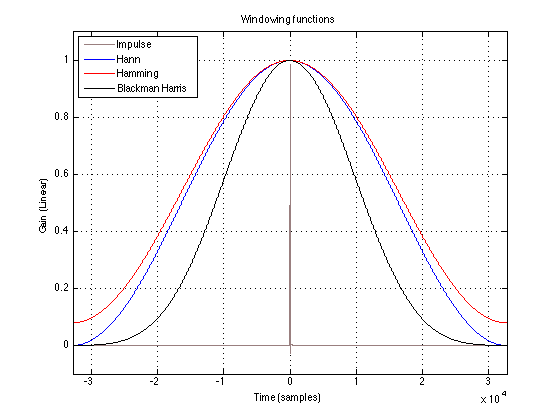Impulse response of an allpass filter with a centre frequency of 100 Hz and three windowing functions with a length of 65536 samples (Fs = 65536 Hz).

Note that the length of the windowing functions is big – 65536 samples to be exact. As you can see in the plot, the ringing of the allpass filter is negligible in this plot by the time we get to the end of the window. This can also be seen below in the next two plots where I’ve shown the impulse response after it has been windowed by the three (actually four, if we include rectangular as a function), scaled in linear and dB FS. (I know, I know, dB FS is an RMS measurement and I plotted this as instantaneous values – sue me.)

So, if you now take those windowed impulse responses and calculate their magnitude and phase responses, you get the plots shown below.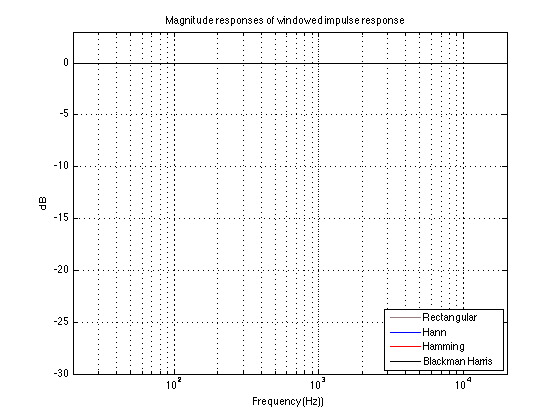Magnitude responses for the windowed impulse responses. Window length = 65536 samples, FFT length = 65536, Fs = 65536. Allpass Fc = 100 Hz.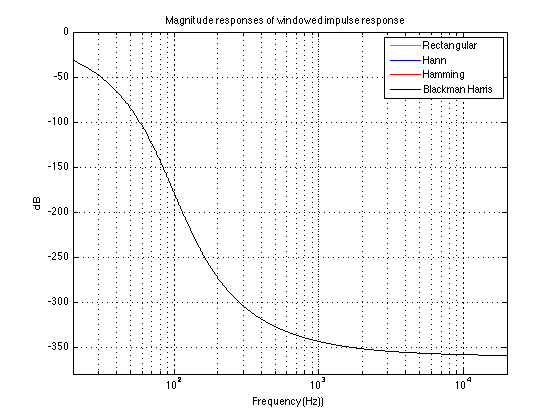Phase responses (in degrees) for the windowed impulse responses. Window length = 65536 samples, FFT length = 65536, Fs = 65536. Allpass Fc = 100 Hz.

“So what?” I hear you cry. The magnitude responses of the four versions of the windowed impulse response are all identical enough that their plots lie on top of each other. This is also true for their phase responses. “I see what I would expect to see – what are you complaining about?” I hear you cry.

Well, let me tell you. The plots above show the results when you use a 65536-point FFT and a 65536-sample window (okay, okay, DFT – sue me).

Let’s do all that again, but with a 65536-point FFT and a 1024-point window instead (I did this in MATLAB, so it’s zero-padding the impulse responses with the remaining 65536-1024 = 64512 samples.)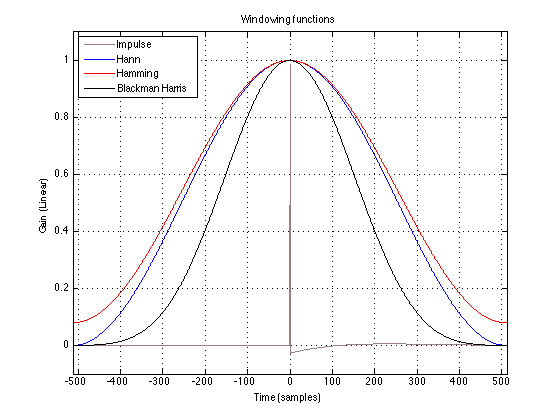Impulse response of an allpass filter with a centre frequency of 100 Hz and three windowing functions with a length of 1024 samples (Fs = 65536 Hz).

Now we can see immediately, that the ringing in the allpass filter’s impulse response hasn’t settled down by the time we get to the end of the window. This can also be seen in the following two plots.

As you can see there, the impulse response itself (aka “Rectangular” windowing) is only about 60 dB below its peak when we reach the end of the window. How does this then affect our magnitude response?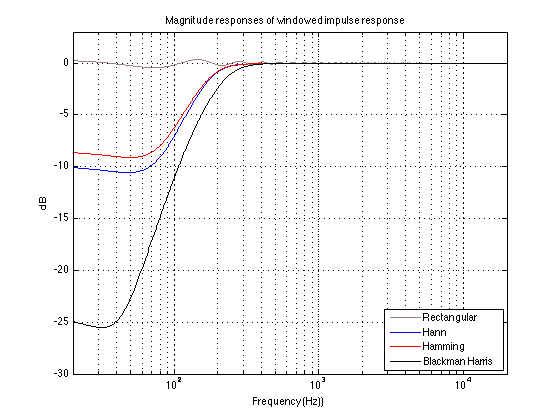Magnitude responses for the windowed impulse responses. Window length = 1024 samples, FFT length = 65536, Fs = 65536. Allpass Fc = 100 Hz.

As you can see there, the implications on the rectangular window is a ripple in the low end of the calculated magnitude response. As you can also see there, the result of attenuating the tail of the allpass filter’s impulse response before we unceremoniously cut it off is that we lose low-end in the magnitude response. The more we attenuate in the windowing function, the more low end we lose.

Of course, this also has implications on the phase response of the windowed impulse responses, as is shown below.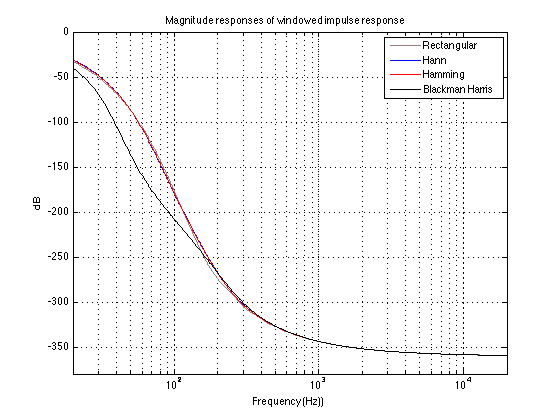Phase responses (in degrees) for the windowed impulse responses. Window length = 1024 samples, FFT length = 65536, Fs = 65536. Allpass Fc = 100 Hz.

The moral of this story is not a new one: beware of the effects of a windowing function on your analysis.

In my personal case, it’s a memorable lesson, since I didn’t get to this conclusion immediately. This is because I was measuring the allpass with different Fc’s – and what I saw in my magnitude response was a shelving response (I was using a Blackman Harris window). When I changed the Fc of the allpass, the shelving response that I saw moved appropriately. So, my conclusion was that there was a problem in my filter that I was measuring. It took some time (too much time!) before I figured out (with the help of some more level-headed friends) that my problem was the window length and my windowing function, not the filter that I was measuring. Won’t make that mistake again for a while…